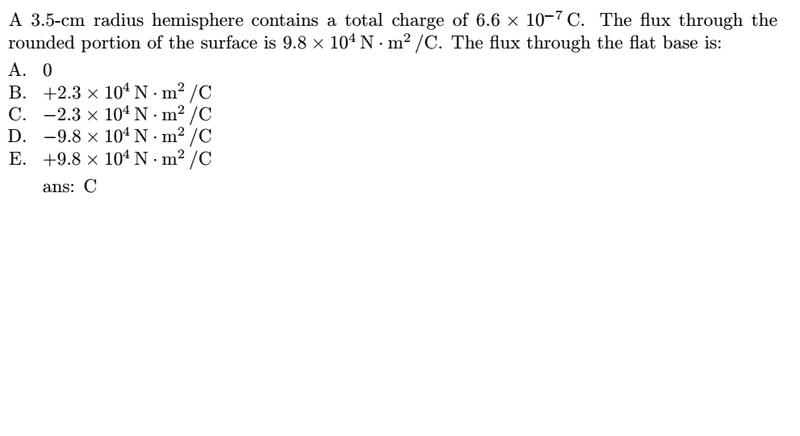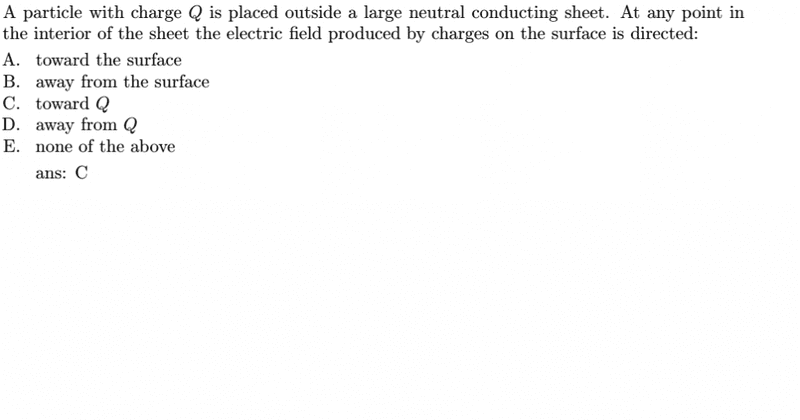# Gauss's law questionI use Gauss's law to calculate: the net electric flux = flux through rounded portion + flux through the flat base = Q/ε. And the flux through the flat base is negative, the negative means?? Help me please, it is very confusing

2)Why they choose C, i think E is correct because Q doesnot identify.

Last edited:

## Answers and Replies

Who help mẹ please :(

Well I guess for the second question it can be because if the charge Q is strong enough it can re-arrange electrons inside the material of the neutral to some extent.

And for the first part, it's because your total flux through the whole hemisphere is roughly 7.45$\times10^{4}$ ,so if the flux through the rounded part is more than that value, the flux through the flat part has to be a negative amount.

Remember this equation, it's quite helpful:

$\Phi_{E}=\oint_{S} \vec{E} \bullet d\vec{S}$

where S is any closed surface

And for the first part, it's because your total flux through the whole hemisphere is roughly 7.45$\times10^{4}$ ,so if the flux through the rounded part is more than that value, the flux through the flat part has to be a negative amount.
I think it is all positive charge so that E is out of it=> no flux through in=> dont exist? why i'm wrong

I think it is all positive charge so that E is out of it=> no flux through in=> dont exist? why i'm wrong

because you are told in the question how the flux is directed. I am not sure how else to explain it, but with the equation stated above your last post, you should be able to calculate your total flux out of the whole geometrical object, which is 7.45×104. So if one portion of the geometrical object has more flux than that value, then the other portion must have a negative amount of flux. (Negative flux means flux into the object, positive flux means directed out from the object)

•1 person
BvU
Science Advisor
Homework Helper
A) The total charge is given, not the distribution. Imagine a dipole +1 microcoulomb close to the top of the hemishpere and -1/3 close to the bottom plate. Fieldlines go out at the top, so positive flux, in at the bottom, negative flux.

B) A bit of a trick question. The field inside the conductor is zero. There are two contribuants: Q and the induced surface charge. First one points away from Q, so the other has to point towards Q.
But I have great difficulty with this answer, since nor Q nor the charges on the surface really produce a field inside the conductor.

But I have great difficulty with this answer, since nor Q nor the charges on the surface really produce a field inside the conductor.

Why that???

SammyS
Staff Emeritus
Science Advisor
Homework Helper
Gold Member
One can look at the field inside the conductor (Yes. It's zero. ) as the superposition of two fields. One field is due to charge Q. The other is due to surface charge on the conducting sheet. The field due to the surface is directed opposite the field due to the Charge Q. However, the problem does not state the sign of charge Q, so we really can't say whether the field due to the surface is directed towards Charge Q or directed away from charge Q.